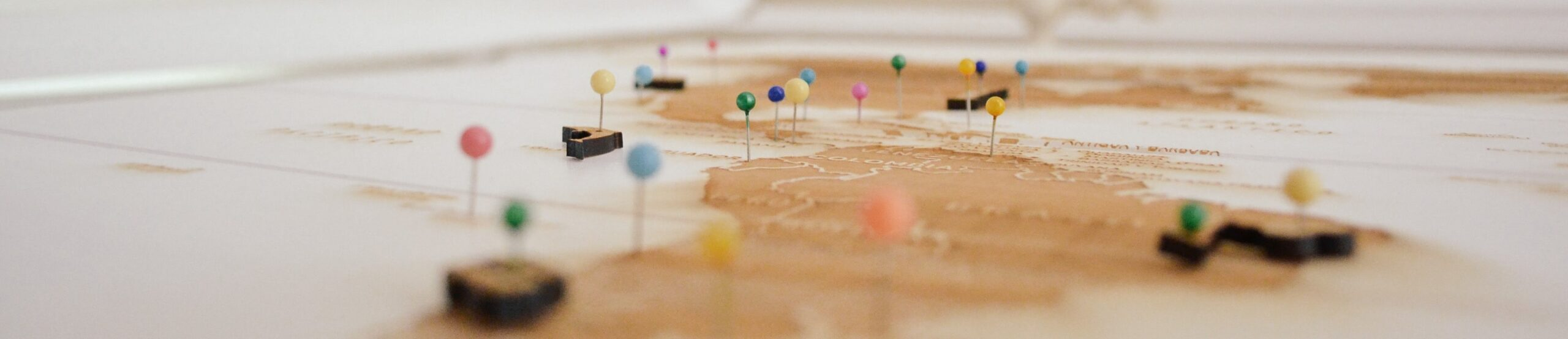#14 Plotting 101: Unravelling the Elements of Plot

#14 Plotting 101: Unravelling the Elements of Plot The Elements of Plot The Elements of plot can be as simple as beginning, middle or end. Alternatively,? you can use complex structures that would make your literary professor salivate. In this article, I share my plot...#13 Plotting 101: An Introduction to Plot

#13 Plotting 101: An Introduction to Plot A Proper Introduction to Plot This article is an introduction to plot and the first of a 5-week series which answers two questions: what is a plot and which elements make up the plot? If you are an aspiring author, use this...